# Day 48 Ring Calibration Latest Results

The software to run the ring calibration is now working

For a "ring" in eta the following examples are the sum of the pedestal subtracted channels, shifted to x=0 with a Chi^2 fit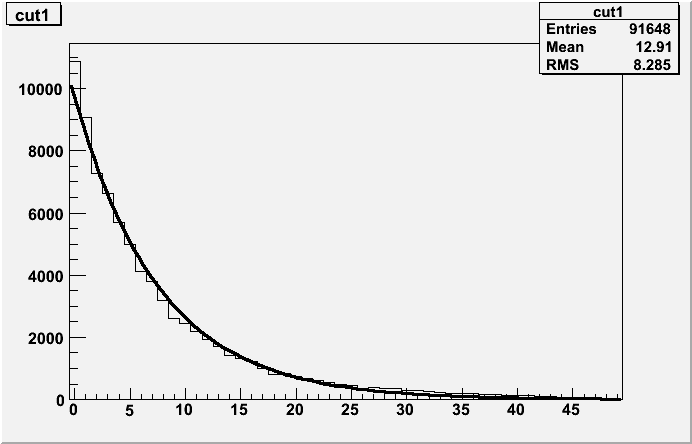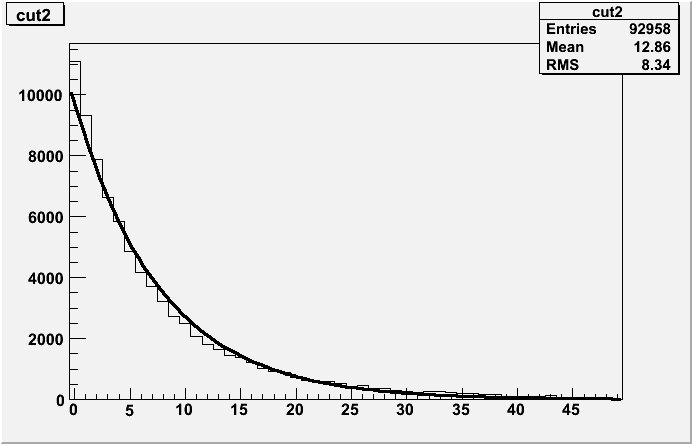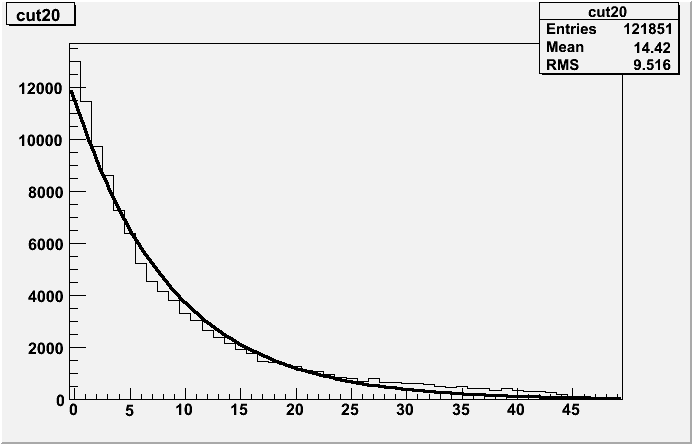For a "ring" in eta the following examples are the sum of the pedestal subtracted channels, shifted to x=0 with a Least Log fit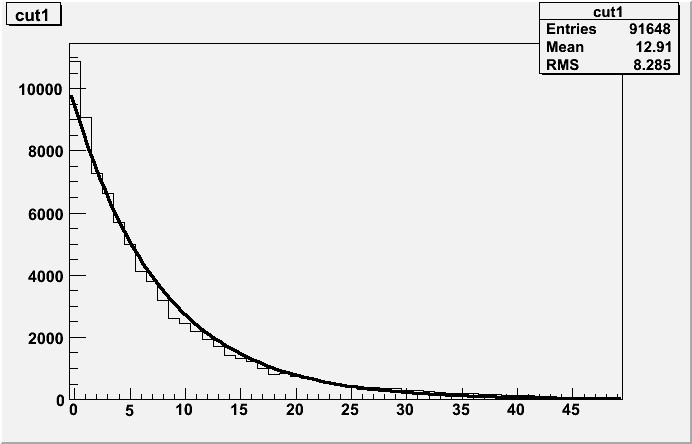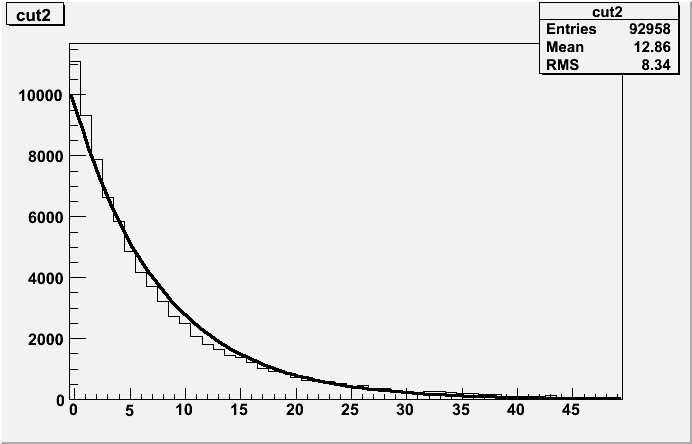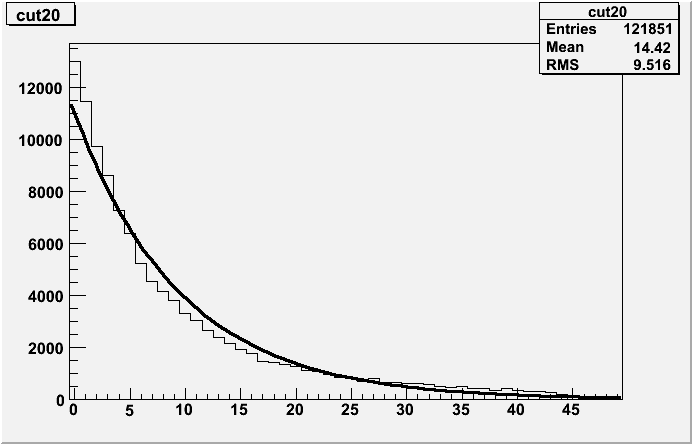For the chi^2 fit, the following plot is the Ring vs. slope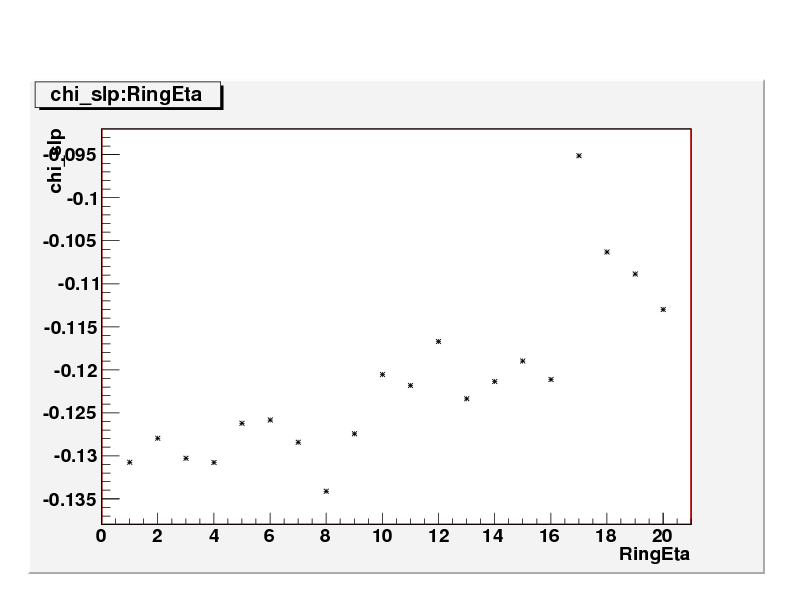Next:

We are running the day 53 data

We are running the p-p data

Calibrate the tower slopes (see previous talks) to these slops for gain

Calibrate to MIPS